## Example Questions

### Example Question #1 : How To Find The Endpoints Of A Line Segment

There is a line defined by two end-points,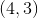and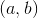.  The midpoint between these two points is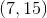.  What is the value of the point?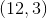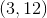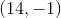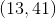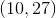Explanation:

Recall that to find the midpoint of two pointsand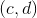, you use the equation: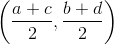.

(It is just like finding the average of the two points, really.)

So, for our equation, we know the following: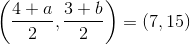You merely need to solve each coordinate for its respective value.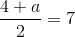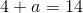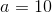Then, for the y-coordinate: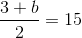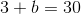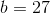Therefore, our other point is:### Example Question #1 : Midpoint Formula

There is a line defined by two end-points,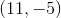and.  The midpoint between these two points is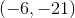.  What is the value of the point?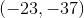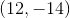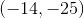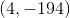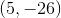Explanation:

Recall that to find the midpoint of two pointsand, you use the equation:.

(It is just like finding the average of the two points, really.)

So, for our equation, we know the following: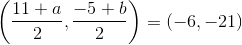You merely need to solve each coordinate for its respective value.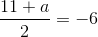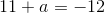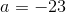Then, for the y-coordinate: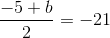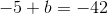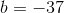Therefore, our other point is:### Example Question #3 : How To Find The Endpoints Of A Line Segment

What is the other endpoint of a line segment with one point that is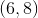and a midpoint of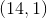?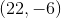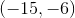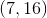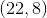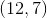Explanation:

Recall that the midpoint formula is like finding the average of theandvalues for two points.  For two pointsand, it is:For our points, we are looking for.  We know: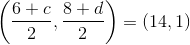We can solve for each of these coordinates separately:

X-Coordinate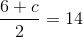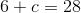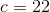Y-Coordinate: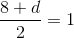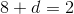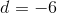Therefore, our point is### Example Question #4 : How To Find The Endpoints Of A Line Segment

What is the other endpoint of a line segment with one point that is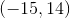and a midpoint of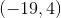?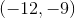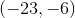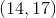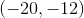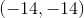Explanation:

What is the other endpoint of a line segment with one point that isand a midpoint of?

Recall that the midpoint formula is like finding the average of theandvalues for two points.  For two pointsand, it is:For our points, we are looking for.  We know: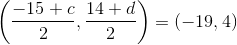We can solve for each of these coordinates separately:

X-Coordinate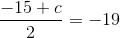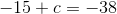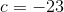Y-Coordinate: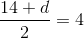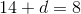Therefore, our point is### Example Question #1 : How To Find The Midpoint Of A Line Segment

What is the midpoint of (2, 5) and (14, 18)?

(16, 23)

(–10, –13)

(7, 9)

(1, 2.5)

(8, 11.5)

(8, 11.5)

Explanation:

The midpoint between two given points is found by solving for the average of each of the correlative coordinates of the given points.  That is:

Midpoint = ( (2 + 14)/2 , (18 + 5)/2) = (16/2, 23/2) = (8, 11.5)

### Example Question #2 : How To Find The Midpoint Of A Line Segment

What is the midpoint between the points (1,3,7) and (–3,1,3)?

(5,2,4)

(3,1,2)

(2,–1,5)

(–1,2,5)

(2,2,5)

(–1,2,5)

Explanation:

To find the midpoint, we add up the corresponding coordinates and divide by 2.

[1 + –3] / 2 = –1

[3 + 1] / 2 = 2

[7 + 3] / 2 = 5

Then the midpoint is (–1,2,5).

### Example Question #71 : Coordinate Geometry

A line which cuts another line segment into two equal parts is called a ___________.

transversal

parallel line

midpoint

horizontal line

bisector

bisector

Explanation:

This is the definition of a bisector.

A midpoint is the point on a line that divides it into two equal parts. The bisector cuts the line at the midpoint, but the midpoint is not a line.

A transversal is a line that cuts across two or more lines that are usually parallel.

Parallel line and horizontal line don't make sense as answer choices here. The answer is bisector.

Tired of practice problems?

Try live online GRE prep today.